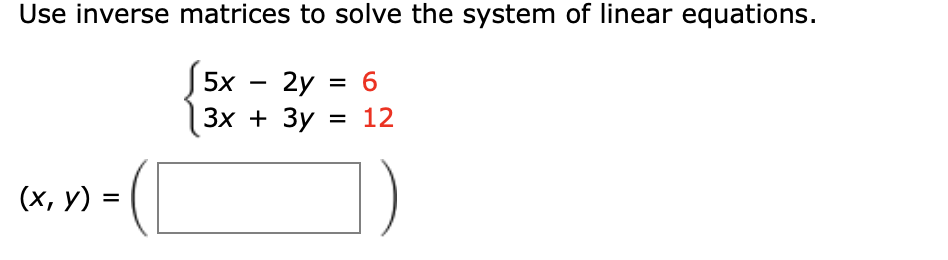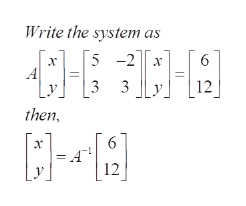# Use inverse matrices to solve the system of linear equations.2yЗх + Зу5x= 612(х, у) 3D

Question
3 viewshelp_outlineImage TranscriptioncloseUse inverse matrices to solve the system of linear equations. 2y Зх + Зу 5x = 6 12 (х, у) 3D fullscreen
check_circle

Step 1

To find the values of x and y satisfying the system using matrix inverse

Step 2

If the given matrix is invertible , then the solution can be found by matrix multiplicationhelp_outlineImage TranscriptioncloseWrite the system as CH 5 -2 A 3 3 12 then 6 12 fullscreen
Step 3

Description of A inverse, if d...

### Want to see the full answer?

See Solution

#### Want to see this answer and more?

Solutions are written by subject experts who are available 24/7. Questions are typically answered within 1 hour.*

See Solution
*Response times may vary by subject and question.
Tagged in

### Math